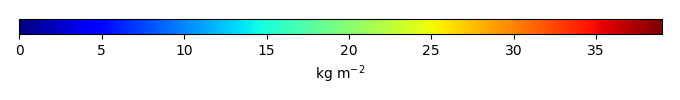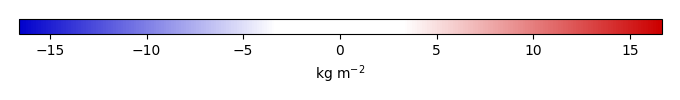# Mean State

Period Mean (original grids) [Pg]
Model Period Mean (intersection) [Pg]
Model Period Mean (complement) [Pg]
Benchmark Period Mean (intersection) [Pg]
Benchmark Period Mean (complement) [Pg]
Bias [kg m-2]
Bias Score 
Spatial Distribution Score 
Overall Score 
Benchmark [-] 30.1
CLM4 [-] 582. 22.9 559. 29.9 0.195 -0.538 0.61 0.77 0.69
CLM4.5 [-] 560. 27.8 532. 29.9 0.195 0.0652 0.60 0.83 0.71
CLM5 [-] 589. 29.0 560. 29.9 0.195 0.319 0.60 0.84 0.72
Period Mean (original grids) [Pg]
Model Period Mean (intersection) [Pg]
Model Period Mean (complement) [Pg]
Benchmark Period Mean (intersection) [Pg]
Benchmark Period Mean (complement) [Pg]
Bias [kg m-2]
Bias Score 
Spatial Distribution Score 
Overall Score 
Benchmark [-] 1.44
CLM4 [-] 4.27 0.825 3.37 1.41 0.0279 -0.354 0.50 0.65 0.57
CLM4.5 [-] 9.20 3.15 5.91 1.41 0.0279 1.69 0.48 0.80 0.64
CLM5 [-] 16.4 5.84 10.4 1.41 0.0279 4.22 0.26 0.35 0.31
Period Mean (original grids) [Pg]
Model Period Mean (intersection) [Pg]
Model Period Mean (complement) [Pg]
Benchmark Period Mean (intersection) [Pg]
Benchmark Period Mean (complement) [Pg]
Bias [kg m-2]
Bias Score 
Spatial Distribution Score 
Overall Score 
Benchmark [-] 0.00649
CLM4 [-] 5.39 0.00905 5.08 0.00649 0.00 0.00778 0.43 0.095 0.26
CLM4.5 [-] 11.4 0.0173 11.1 0.00649 0.00 0.148 0.56 0.79 0.68
CLM5 [-] 11.9 0.0238 11.6 0.00649 0.00 0.192 0.54 0.67 0.61
Period Mean (original grids) [Pg]
Model Period Mean (intersection) [Pg]
Model Period Mean (complement) [Pg]
Benchmark Period Mean (intersection) [Pg]
Benchmark Period Mean (complement) [Pg]
Bias [kg m-2]
Bias Score 
Spatial Distribution Score 
Overall Score 
Benchmark [-] 17.4
CLM4 [-] 16.4 16.0 0.277 17.3 0.0180 -0.0107 0.67 0.91 0.79
CLM4.5 [-] 20.4 19.9 0.343 17.3 0.0180 1.01 0.68 0.91 0.80
CLM5 [-] 17.8 17.3 0.314 17.3 0.0180 0.419 0.69 0.92 0.80
Period Mean (original grids) [Pg]
Model Period Mean (intersection) [Pg]
Model Period Mean (complement) [Pg]
Benchmark Period Mean (intersection) [Pg]
Benchmark Period Mean (complement) [Pg]
Bias [kg m-2]
Bias Score 
Spatial Distribution Score 
Overall Score 
Benchmark [-] 1.52
CLM4 [-] 25.1 1.02 24.2 1.46 0.0621 -0.890 0.48 0.68 0.58
CLM4.5 [-] 34.8 1.47 33.5 1.46 0.0621 1.42 0.60 0.76 0.68
CLM5 [-] 56.8 2.46 54.5 1.46 0.0621 7.01 0.56 0.88 0.72
Period Mean (original grids) [Pg]
Model Period Mean (intersection) [Pg]
Model Period Mean (complement) [Pg]
Benchmark Period Mean (intersection) [Pg]
Benchmark Period Mean (complement) [Pg]
Bias [kg m-2]
Bias Score 
Spatial Distribution Score 
Overall Score 
Benchmark [-] 9.78
CLM4 [-] 5.11 4.98 0.169 9.71 0.0683 -1.19 0.55 0.42 0.48
CLM4.5 [-] 3.42 3.26 0.205 9.71 0.0683 -1.67 0.47 0.26 0.37
CLM5 [-] 3.49 3.35 0.157 9.71 0.0683 -1.61 0.49 0.57 0.53

# Temporally integrated period mean

BENCHMARK MEANMODEL MEANBIASBIAS SCORESPATIAL TAYLOR DIAGRAMMODEL COLORS# Spatially integrated regional mean

MODEL COLORS# All Models

BenchmarkCLM4CLM4.5CLM5